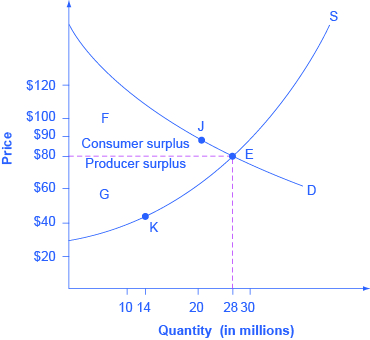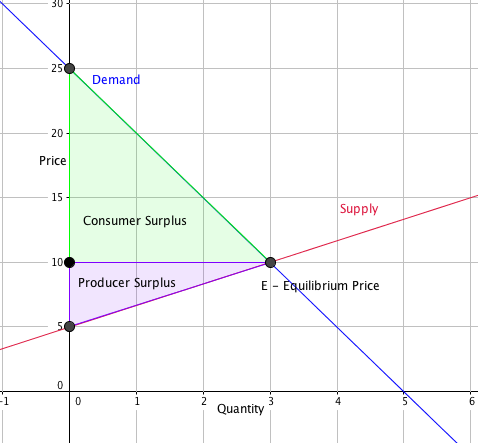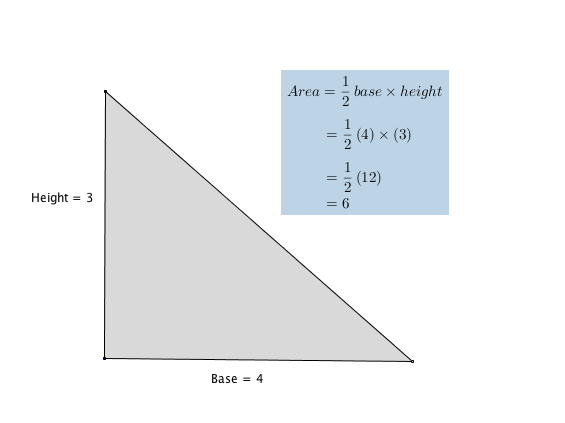## Demand, Supply and Efficiency

The familiar demand and supply diagram holds within it the concept of economic efficiency. One typical way that economists define efficiency is when it is impossible to improve the situation of one party without imposing a cost on another. Conversely, if a situation is inefficient, it becomes possible to benefit at least one party without imposing costs on others.

Efficiency in the demand and supply model has the same basic meaning: The economy is getting as much benefit as possible from its scarce resources and all the possible gains from trade have been achieved. In other words, the optimal amount of each good and service is being produced and consumed.

## Consumer Surplus, Producer Surplus, Social Surplus

Consider a market for tablet computers, as shown in Figure 1. The equilibrium price is $80 and the equilibrium quantity is 28 million. To see the benefits to consumers, look at the segment of the demand curve above the equilibrium point and to the left. This portion of the demand curve shows that at least some demanders would have been willing to pay more than$80 for a tablet.Figure 1: Consumer and Producer Surplus

For example, point J shows that if the price was $90, 20 million tablets would be sold. Those consumers who would have been willing to pay$90 for a tablet based on the utility they expect to receive from it, but who were able to pay the equilibrium price of $80, clearly received a benefit beyond what they had to pay for. Remember, the demand curve traces consumers’ willingness to pay for different quantities. The amount that individuals would have been willing to pay, minus the amount that they actually paid, is called consumer surplus. Consumer surplus is the area labeled F—that is, the area above the market price and below the demand curve. The somewhat triangular area labeled by F in the graph above shows the area of consumer surplus, which shows that the equilibrium price in the market was less than what many of the consumers were willing to pay. Point J on the demand curve shows that, even at the price of$90, consumers would have been willing to purchase a quantity of 20 million. The somewhat triangular area labeled by G shows the area of producer surplus, which shows that the equilibrium price received in the market was more than what many of the producers were willing to accept for their products. For example, point K on the supply curve shows that at a price of $45, firms would have been willing to supply a quantity of 14 million. The supply curve shows the quantity that firms are willing to supply at each price. For example, point K in Figure 1 illustrates that, at$45, firms would still have been willing to supply a quantity of 14 million. Those producers who would have been willing to supply the tablets at $45, but who were instead able to charge the equilibrium price of$80, clearly received an extra benefit beyond what they required to supply the product. The amount that a seller is paid for a good minus the seller’s actual cost is called producer surplus. In Figure 1, producer surplus is the area labeled G—that is, the area between the market price and the segment of the supply curve below the equilibrium.

Example: Calculate consumer surplus given linear supply and demand curves.In the sample market shown in the graph, equilibrium price is $10 and equilibrium quantity is 3 units. The consumer surplus area is highlighted above the equilibrium price line. This area can be calculated as the area of a triangle. Recall that to find the area of a triangle, you will need to know it’s base and height. Refer to the following example if you need a refresher.Let’s apply the calculation for the area of a triangle to our example market to see the added value that consumers will get for this item at the equilibrium price in our sample market. Setp 1 Define the base and height of the consumer surplus triangle. The base of the consumer surplus triangle is 3 units long. Be careful when you define the height of this triangle, it is tempting to say it is 25, can you see why it isn’t? The height is determined by the distance from the equilibrium price line and where the demand curve intersects the vertical axis. The height of the triangle begins at$10 and ends at $25, so it will be$25 – $10 =$15

$b = 3$

$h = 15$

Setp 2 Apply the values for base and height to the formula for the area of a triangle.

A = $\frac{1}{2}b\times h$

A = $\frac{1}{2}3\times 15$

A = $\frac{1}{2}45$

A = $\frac{45}{2} = 22.5$

By calculating the consumer surplus value, we can gain insight into the price elasticity of supply and demand. When demand is inelastic, consumer surplus is greater. Can you explain why? How would consumer surplus change as market price changes?

The sum of consumer surplus and producer surplus is social surplus, also referred to as economic surplus or total surplus. In Figure 1, social surplus would be shown as the area F + G. Social surplus is larger at equilibrium quantity and price than it would be at any other quantity. This demonstrates the economic efficiency of the market equilibrium. In addition, at the efficient level of output, it is impossible to produce greater consumer surplus without reducing producer surplus, and it is impossible to produce greater producer surplus without reducing consumer surplus.

## Self Check: Consumer Surplus

Answer the question(s) below to see how well you understand the topics covered in the previous section. This short quiz does not count toward your grade in the class, and you can retake it an unlimited number of times.

You’ll have more success on the Self Check if you’ve completed the Reading in this section.

Use this quiz to check your understanding and decide whether to (1) study the previous section further or (2) move on to the next section.